# Tutorial: Open a model to browse

Release: 4.6  •  5.0  •  5.1  •  5.2  •  5.3  •  5.4  •  6.0  •  6.1  •  6.2  •  6.3  •  6.4

This Tutorial page shows you how to open and explore an example Analytica model: The Rent vs. Buy model compares the total cost of renting a house to the cost of buying one.

You can start by viewing this video, or jump straight into starting up the model in Analytica.

Video: Tutorial: Open a model to browse (8 minutes)

## Open the Rent vs. Buy model

1. You start Analytica like any Windows application: For example, click the Start button on the Windows taskbar. Click All ProgramsAnalytica 6.3Analytica 6.3.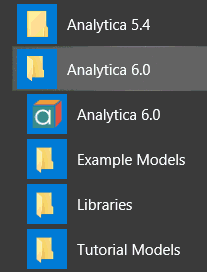2. After Analytica starts, select the Rent vs. Buy Example on the Intro screen.

## The Diagram window

When you open a model, Analytica first displays a top-level Diagram window. A diagram is a graphical user interface that displays user inputs and outputs, influence diagrams, pictures, and other visual elements and controls, and is designed by the author of the model. The Rent vs. Buy model diagram shows several input variables that affect the trade-offs between renting and buying, Normal distribution buttons, a Calc button, and a node labeled Model.

Normal distrib. button

User input

Model node

Calc button to see results

This top-level diagram is an end-user interface to the model itself, which is contained in the Model node. In this chapter, you use only the interface in this top level diagram; in the following chapters you will explore the model in more depth.

Across the top of the screen is a horizontal palette of buttons. This is called the tools palette.

When you first open the Rent vs. Buy model, the browse tool is highlighted on the palette. With the browse tool selected, the cursor looks like a hand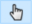when it is over the diagram. The browse tool allows you to calculate the model, change input values, and examine — but not change — the structure of the model. In this chapter, you only use the browse tool.

## Access Help Resources

At any time, you can press the F1 key on the keyboard or use the Help menu to access Analytica’s help resources online. This menu includes links to this Tutorial, the User Guide and much more tips and reference material on this Analytica Wiki.

## Compute output values

In the Rent vs. Buy model, the output value of interest is at the bottom, Present value of buying and renting.

1.  Click the Calc button to compare the present value of buying and renting.

The output value displays in a Result window. This Result window shows a graph of two probability density curves, one for buying and one for renting. In a probability density graph, the units of the vertical scale are chosen so that the total area under each curve is 1 (100%). 25μ corresponds to 25 x 10-6 or 0.000025.

Tip
Numerical suffixes like μ and K are used extensively throughout Analytica. A quick reference for these suffixes is given in Number formats .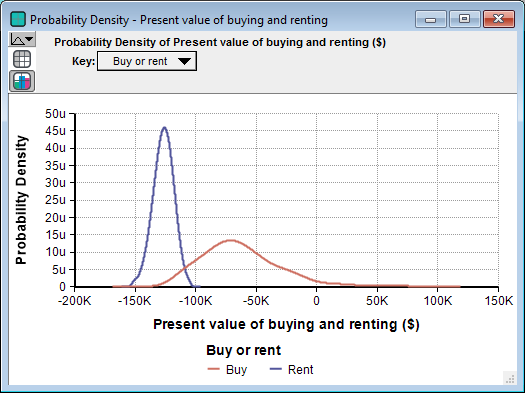Since the graph is of probability densities, both buying and renting have probabilistic, or uncertain, inputs. The probability density graph for each appear to be bell-shaped curves (normal distribution), although they appear a bit “noisy.”

The graphs show that the cost of renting, given the model’s inputs, are between about $105,000 and$155,000 (the negative numbers mean cost — cash flowing out), while the cost of buying is between $115,000 and a gain of$75,000.

2.  Click the Diagram window to bring it to the front.

Note: Your results can vary slightly, since the model is generating random inputs based on a normal distribution for the uncertainty of the rate of inflation and for the appreciation rate.

Click the model Diagram window to bring it to the front. Notice that the button next to Costs of buying and renting has changed to Result. The Result button indicates that the value has been computed; clicking the Result button re-displays the computed values.

## Examine definition types in user input nodes

When you enter a value into a user input, you need to enter a value that is compatible with it's definition type. See more here...Expression popup menu.

Click the Time horizon node.
Press F4 to bring up the object window.

1.  Click the Variable Time horizon, then press F4.

Click on the Expression popup menu (here it has a numeric icon, since this input is restricted to numbers only).
Note that Number only is selected. If you attempt enter text into the input node you will get a warning.

2.  Click on the Expression popup menu - Note that Number only is selected.

Close the object window - click the object window icon at the top left of the object window and select Close from the popup menu.

3.  Click on the Object window icon.

4.  Select Close.

## Change input values and recompute results

Now you will change some input values to the model and recompute the rent vs. buy comparison. You will change the values of Time horizon, Monthly rent, and Buying price.

1.  Click the box next to Time horizon. Change the value to 7 and press Alt+Enter.
Tip
The main Enter key and the numeric keypad Enter key are not interchangeable. They have different functions in Analytica. Alt+Enter is equivalent to the numeric keypad Enter key.

As soon as you change an input, the Result button changes to a Calc button, indicating that Present value of buying and renting needs to be recomputed.

2.  Click the box next to Monthly rent. Change the value to 1400 and press Alt+Enter.

3.  Click the box next to Buying price. Change the value to 180K (or 180000) and press Alt+Enter.

Now you are ready to recompute to see the new results.

4.  Click the Calc button to compute the comparison of the costs of buying to renting.

The graphs show that the cost of renting, given these changed inputs, is between $90,000 and$120,000, while the cost of buying is between $135,000 and a gain of$70,000.

5.  Click the Diagram window to bring it to the front.

## Examine and change an uncertain input

When an input is defined as a probability distribution, a button with the name of the distribution appears next to the input’s name. Clicking this button opens the Object Finder window, in which you can see details and change the distribution’s parameters or type of distribution.

Rate of inflation’s button says Normal, indicating that it is defined as a normal distribution.

1.  Click the Normal button next to the Rate of inflation.

The Object Finder window appears. It shows that Rate of inflation is defined as a normal distribution with a mean of 3.5 and a standard deviation of 1.3.

You will now modify the probability distribution that defines Rate of inflation. Rather than using the normal distribution, you will use the uniform distribution, and assume that inflation has an equal probability of being anywhere between 3% and 4% per year.

2.  Scroll down the list of distributions and select Uniform

3.  Change the minimum to 3.

4.  Change the maximum to 4.

5.  Click OK to accept the change.

6.  Click the Calc button to compute the new comparison of the present value of buying to renting.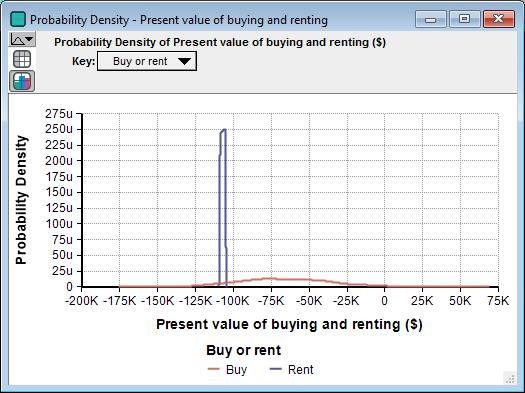The graphs show that the uncertainty in the cost of renting has narrowed to between about $105,000 and$109,000, while the uncertainty in the cost of buying has flattened to between about $125,000 and a gain of$10,000.

## Display alternative uncertainty views

Analytica offers a variety of views to display uncertain values, including selected statistics, probability bands, the probability density function, the cumulative probability distribution function, measures of central tendency, and the table of random numbers from which the uncertain distribution is estimated.

You will now examine several of these views.

In the upper-left corner of the Result window is the Uncertainty View popup menu.The miniature probability distribution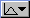indicates that Probability Density is selected.

1.  Press on the Uncertainty view popup menu and select Cumulative probability.

The Result window now shows two cumulative probability curves. Along the vertical axis, these curves give the probability that each cost is less than a given value along the horizontal axis.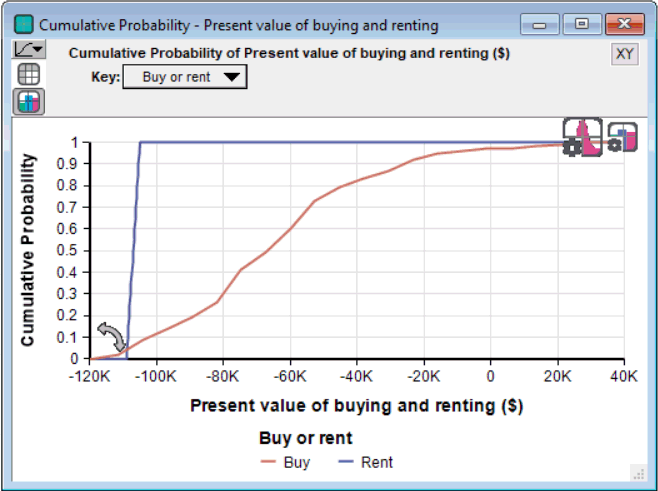There appears to be about a 50% probability that the cost to buy is below $70,000, while the cost to rent has a 50% probability of being below about$110,000.

Sometimes you might want to see an uncertain value expressed as a single number — a measure of central tendency. Analytica computes the mid value (sometimes called the deterministic value) by fixing all input probability distributions at their median (50% probability) values. The mid value is the only uncertainty view available for nonprobabilistic results.

2.  Select Mid Value""" from the Uncertainty view popup menu.

The Result window now displays bar graphs for the two mid values.

Under the Uncertainty View popup menu are two buttons,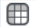and. Theis highlighted, indicating that the Result window is displaying a graph view. The Result window can also display numeric values in a spreadsheet-like table view.Analytica also provides the mean (or average) value.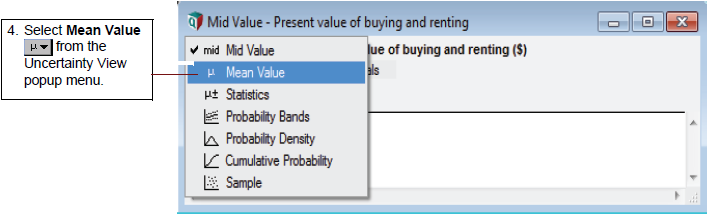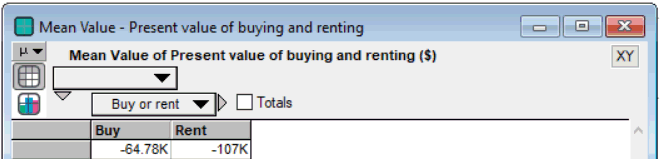You can also view a set of statistics, including both the median and mean, the ranges (minimum and maximum), and the standard deviation.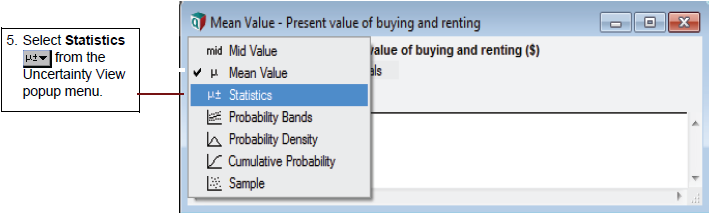The Result window now displays the minimum, median, mean, maximum, and standard deviation for Costs of buying and renting.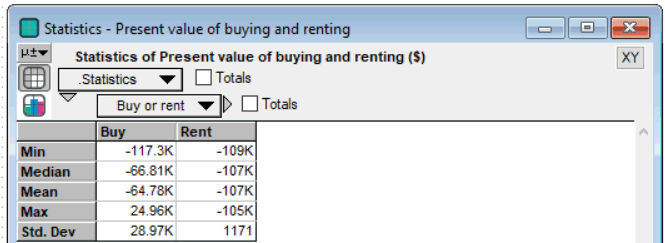The statistics might not be exact, because they are estimated from a sample of values from the distribution.

Finally, you see the sample values.The table above lists the 100 sample values that Analytica randomly generated from the probability distribution to estimate the statistics.

A sample size of 100 is adequate for most applications; however, if you need more precise estimates, you can increase the sample size. See Uncertainty Setup dialog in the Analytica User Guide.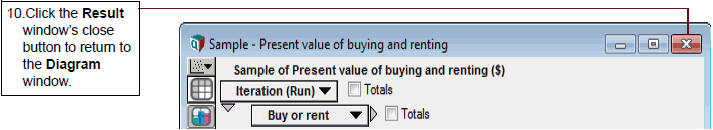If you want to save changes to your model, you can do so at this point. (For instructions on quitting without saving, see the next section.

If you wish to save your model as a different file, so that you do not change the original model, select Save As from the File menu.

## Quit Analytica

When you have finished using a model, you might want to quit Analytica.

## Summary

You have now opened an Analytica model, calculated and viewed the results, changes input values and probability distributions, and displayed the uncertain results in several ways. These are the basic techniques for using any quantitative model.

After you create your own models, you might want to give them a top-level input and output diagram like the one used in this chapter. For information about customizing a model for end users, see Creating Interfaces for End Users in the Analytica User Guide.

The next Tutorial page, shows how to navigate the details of the Rent vs. Buy model, exploring its structure and contents.